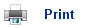# Light, Spectra and Electrons

• Most of our knowledge of the atomic structure has come from the area of science known as spectroscopy- the study of how light and matter interact.
• Chemists use two theories to describe light. The wave theory and the particle theory. Both can be used, depending on which property of light is being referred to. That is, the method which best describes the situation is chosen.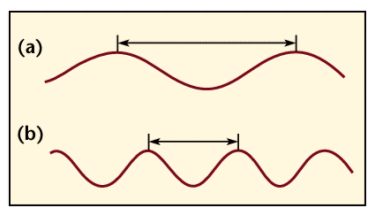• Wavelength is the measure of distance travelled by the wave in one cycle. The frequency is how many cycles the wave goes through every second.
• Wave “a” has a longer wavelength than “b”, but because they both travel at the speed of light; the frequency of “b” is twice that of “a”.
• This can be summarised as: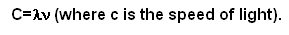• So if the wavelength is halved the frequency is doubled, and vice-versa.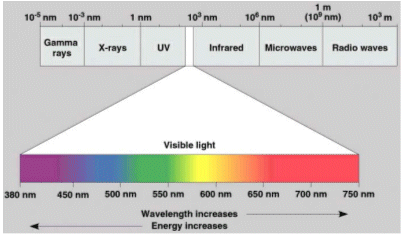## The particle theory of light

• In some situations, light can be best described using the particle theory.
• The theory was proposed by Albert Einstein in 1905, and it regards light as a stream of energy called “photons”. The energy of the photons, determines whereabouts the light is in the electromagnetic spectrum.
• Both the wave theory and the particle theory are linked by a formula: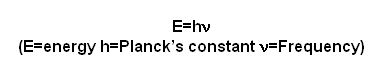• The energy contained in the photon is equal to the frequency of the radiation multiplied by Planck’s constant 6.63x10-34JHz-1.
• For a photon of infrared ray with energy of 6x10-20J, the frequency could be worked out by dividing the energy by Planck’s constant. So 6x10-20/6.63x10-34JHz-1= 9x1013Hz.

## Energy levels

• If an atom is given energy, ionisation can take place; this is where the atom loses an electron.
• If there is not enough energy to ionise the atom, there may be enough to “shift” the electrons up an energy level. This is called promotion.
• A promotion to another energy level requires a specific energy. When the electrons move back down a level (demotion) they emit this specific energy as light.
• The ionisation energy, and the promotion energy required can be worked out by the light that is emitted (the energy can be worked out by multiplying the frequency of light by Planck’s constant).

## Useful books for revision:

Revise AS Chemistry for Salters (Written by experienced examiners and teachers of Salter's chemistry)Revise AS Chemistry for Salters (OCR) (Salters Advanced Chemistry)Home